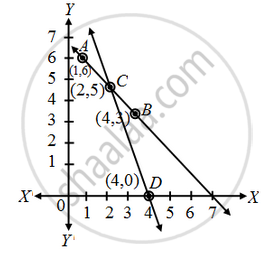# The path of highway number 1 is given by the equation x + y = 7 and the highway number 2 is given by the equation 5x + 2y = 20. Represent these equations geometrically - Mathematics

Sum

The path of highway number 1 is given by the equation x + y = 7 and the highway number 2 is given by the equation 5x + 2y = 20. Represent these equations geometrically

#### Solution

We have, x + y = 7

⇒ y = 7 – x ….(1)

In tabular form

 x 1 4 y 6 3 points A B

and 5x + 2y = 20

⇒ y = \frac { 20-5x }{ 2 } ….(2)

In tabular form

 x 2 4 y 5 0 points C D

Plot the points A (1, 6), B(4, 3) and join them to form a line AB.

Similarly, plot the points C(2, 5). D (4, 0) and join them to get a line CD. Clearly, the two lines intersect at the point C. Now, every point on the line AB gives us a solution of equation (1). Every point on CD gives us a solution of equation (2)Concept: Graphical Method of Solution of a Pair of Linear Equations
Is there an error in this question or solution?